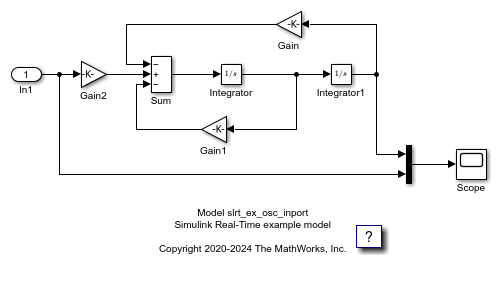# Stimulate Root Inport by Using MATLAB Language

This example shows how to stimulate root inports in a model by using the Stimulation object and related functions:

• start

• stop

• getStatus

• pause

### Open Model and Map Inport to Wave Data

Open model `slrt_ex_osc_inport`. Save the model to a working folder. Map the inport to use square wave data. For inport `In1`, interpolated is off.

```model = ('slrt_ex_osc_inport'); open_system(model); load(fullfile(matlabroot,'toolbox','slrealtime','examples','slrt_ex_inport_square.mat')); waveform = square; set_param(model,'ExternalInput','waveform'); set_param(model,'LoadExternalInput','on'); set_param(model,'StopTime','Inf'); ``````evalc('slbuild(model)'); tg = slrealtime('TargetPC1'); load(tg,model); ```

### Stimulate Root Inport Data

Start root inport stimulation of inports 1. Open Scope block and observe results.

```start(tg.Stimulation,); start(tg); ```

Pause root inport stimulation of inport 1.

```pause(tg.Stimulation,); ```

Stop and start the stimulation of inport 1.

```stop(tg.Stimulation,); start(tg.Stimulation,); ```

Check the status of stimulation of the inports.

```getStatus(tg.Stimulation,'all'); ```

Create a time-series object to load data to an inport.

```sampleTime = 0.1; endTime = 10; numberOfSamples = endTime * 1/sampleTime + 1; timeVector = (0:numberOfSamples) * sampleTime; u = timeseries(timeVector*10,timeVector); ```

Object u is created for 10 seconds. Load it to the inport 1. Stimulation of an inport should be stopped before loading data.

```stop(tg.Stimulation,); reloadData(tg.Stimulation,,u); ```

Stop real-time application and close all.

```stop(tg); bdclose('all'); ```